# Introduction / examples

## Definition

A system is said to be nonlinear when one of the properties of the system is a function of the variable that is an unknown of the system. Two examples are presented in the following paragraph.

## Example 1 (thermal application)

The differential equation solved using finite element method in a Steady State Thermal application is the following: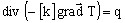where:

• [k] is the tensor of thermal conductivity
• q is the volume density of power of the heat source
• T is the temperature, respectively the state variable, i.e. the unknown of the system.

If the thermal conductivity k is a function of the temperature T, the system is a nonlinear system.

## Example 2 (magnetic application)

The differential equation solved using the finite element method in a Magneto Static application (with the scalar model) can be written: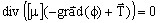where:

• [μ] is the tensor of magnetic permeability in the computation domain
• ϕ is a magnetic scalar potential, respectively the state variable
•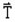is a term corresponding to sources (imposed field source or electric vector potential).

If the magnetic permeability μ is a function of the magnetic field H, respectively of the state variable ϕ, the system is a nonlinear system.

## Example 2 prime (magnetic application)

The differential equation solved using finite element method in a Magneto Static application (with vector model) can be written: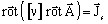where:

• [ν] is the tensor of magnetic reluctivity of the computation domain
•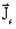is the density of current source
•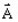is the vector potential, respectively the state variable, i.e. the unknown of the system

If the magnetic reluctivity ν is a function of the magnetic flux density B, respectively of the state variable A, the system is a nonlinear system.

## Different possibilities

A system is called nonlinear in Flux applications when:

• behavior laws of materials (constitutive equations) are nonlinear
• B(H) nonlinear law: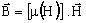(magnetic permeability μ function of the magnetic field H)

• J(E) nonlinear law: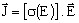(electric conductivity σ function of the electric field E)

• D(E) nonlinear law: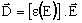(electric permitttivity ε function of the electric field E)

• a thermal property depends on temperature…
• thermal conductivity k function of T
• volume heat capacity function of T
• thermal exchange coefficients function of T

## ... in Flux

A brief description of the models proposed in Flux is presented in the two tables below:
• Models for the behavior laws (electromagnetic properties)
B(H) J(E) D(E)
Soft materials Hard materials
Linear Linear Linear Constant resistivity Linear
Linear complex

Linear complex

Linear with losses

Nonlinear

Saturation:

• analytic
• spline

Rayleigh

Demagnetization:

• analytic